# TensorFlow新特性实战开发：构建强大的机器学习模型

## TensorFlow新特性实战开发：构建强大的机器学习模型

TensorFlow新特性实战开发：构建强大的机器学习模型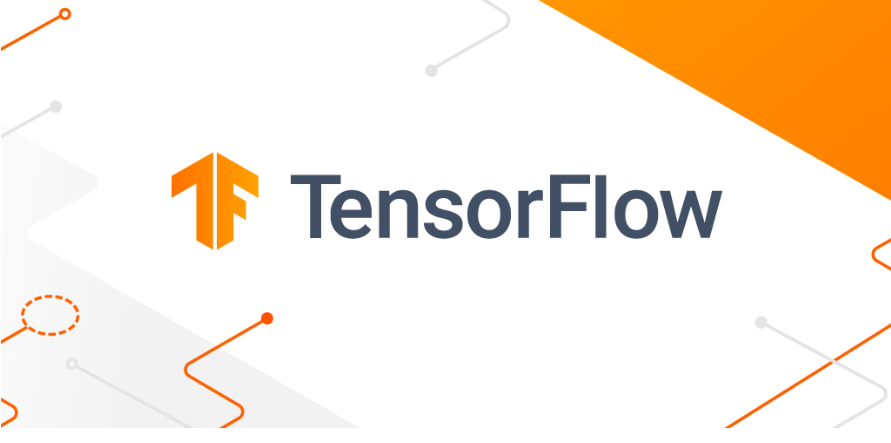### 二 . 数据准备

``````import tensorflow as tf
​
# 加载图像数据集
dataset = tf.keras.preprocessing.image_dataset_from_directory(
'path/to/dataset',
batch_size=32,
image_size=(128, 128),
validation_split=0.2,
subset='training'
)
​
# 数据增强
data_augmentation = tf.keras.Sequential([
tf.keras.layers.experimental.preprocessing.RandomFlip('horizontal'),
tf.keras.layers.experimental.preprocessing.RandomRotation(0.2),
])
​
# 对数据集应用数据增强
augmented_dataset = dataset.map(lambda x, y: (data_augmentation(x, training=True), y))
​``````

### 三 . 模型构建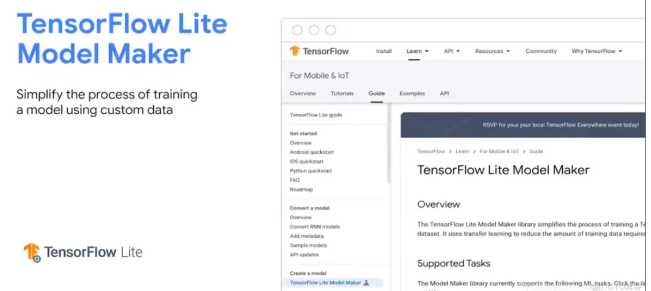TensorFlow提供了多种构建模型的方法，包括使用Sequential模型、函数式API和自定义模型。我们可以根据任务需求和模型复杂度选择合适的方法。以下是使用Sequential模型构建一个简单的卷积神经网络（CNN）的示例代码：

``````import tensorflow as tf
​
model = tf.keras.Sequential([
tf.keras.layers.Conv2D(32, (3, 3), activation='relu', input_shape=(32, 32, 3)),
tf.keras.layers.MaxPooling2D((2, 2)),
tf.keras.layers.Flatten(),
tf.keras.layers.Dense(64, activation='relu'),
tf.keras.layers.Dense(10, activation='softmax')
])
​``````

### 四. 模型训练与优化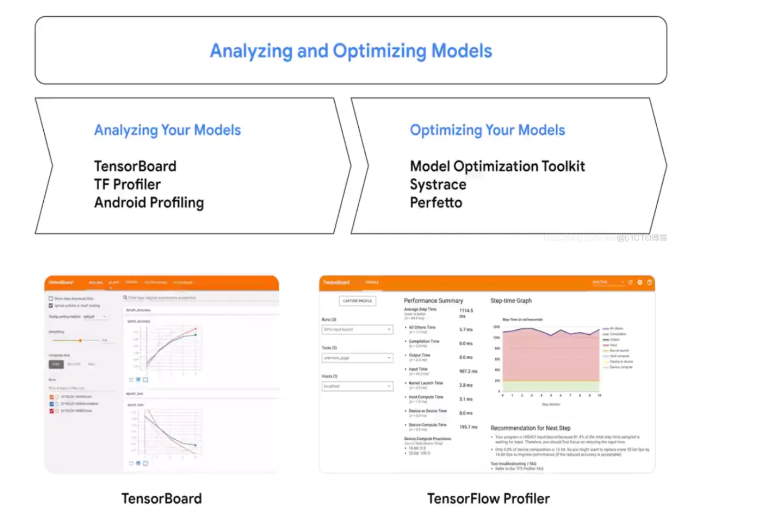``````model.compile(optimizer='sgd',
loss='mean_squared_error',
metrics=['accuracy'])
​``````

``````model.fit(train_dataset,
epochs=10,
validation_data=val_dataset)``````

### ​​​​​​​五. 模型评估和部署、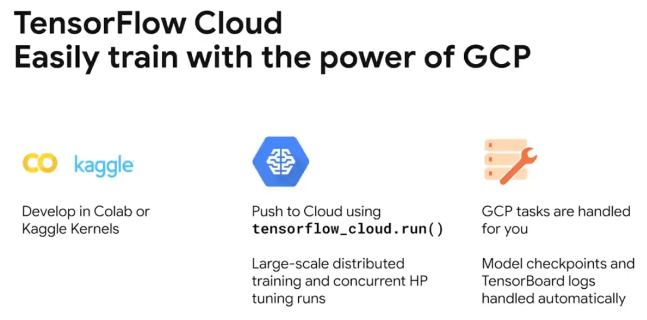```test_loss, test_accuracy = model.evaluate(test_dataset)
print('Test Loss:', test_loss)
print('Test Accuracy:', test_accuracy)
​```

### 六 .TensorFlow生态系统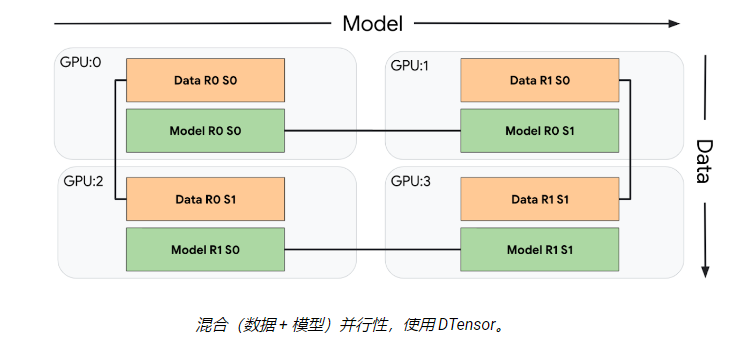TensorFlow生态系统 TensorFlow拥有一个庞大而丰富的生态系统，提供了许多扩展工具和库来支持机器学习开发。以下是一些TensorFlow生态系统中常用的组件和库：

• TensorFlow Hub：提供了预训练模型和模型组件，可以方便地在自己的模型中使用预训练好的权重和结构。

• TensorFlow Extended（TFX）：是一个端到端的机器学习平台，用于支持生产环境中的机器学习工作流程，包括数据准备、模型训练、模型评估和部署等。

• TensorFlow.js：是一个用于在浏览器中运行机器学习模型的库，使得在Web应用中实现机器学习变得更加便捷。

• TensorFlow on Spark：将TensorFlow与Apache Spark相结合，实现分布式计算和大规模机器学习。

...全文
28 回复 打赏 收藏 转发到动态 举报TensorFlow机器学习系统-其他
TensorFlow是用于机器学习的端到端开源平台。它拥有一个全面而灵活的生态系统，其中包含各种工具、库和社区资源，可助力研究人员推动先进机器学习技术的发展，并使开发者能够轻松地构建和部署由机器学习提供支持的应用。 TensorFlow最初是由Google机器智能研究组织内Google Brain团队的研究人员和工程师开发的，用于进行机器学习和深度神经网络研究。该系统具有足够的通用性，也可以适用于多种其他领域。 TensorFlow提供稳定的Python和C++ API，以及其他语言的非保证向后兼容API 。 轻松地构建模型 TensorFlow提供多个抽象级别，因此您可以根据自己的需求选择合适的级别。您可以使用高阶Keras API构建和训练模型，该API让您能够轻松地开始使用TensorFlow机器学习。 如果您需要更高的灵活性，则可以借助即刻执行环境进行快速迭代和直观的调试。对于大型机器学习训练任务，您可以使用Distribution Strategy API在不同的硬件配置上进行分布式训练，而无需更改模型定义。 随时随地进行可靠的机器学习生产 TensorFlow始终提供直接的生产途径。不管是在服务器、边缘设备还是网络上，TensorFlow都可以助您轻松地训练和部署模型，无论您使用何种语言或平台。 如果您需要完整的生产型机器学习流水线，请使用TensorFlow Extended (TFX)。要在移动设备和边缘设备上进行推断，请使用TensorFlow Lite。请使用TensorFlow.js在JavaScript环境中训练和部署模型强大的研究实验 构建和训练先进的模型，并且不会降低速度或性能。借助Keras Functional API和Model Subclassing API等功能，TensorFlow可以助您灵活地创建复杂拓扑并实现相关控制。为了轻松地设计原型并快速进行调试，请使用即刻执行环境。 TensorFlow还支持强大的附加库和模型生态系统以供您开展实验，包括Ragged Tensors、TensorFlow Probability、Tensor2Tensor和BERT。tensorflow有哪些常用的函数
TensorFlow 是一个强大机器学习框架，具有大量的内置函数，以便于构建、训练和评估机器学习模型。以下是 TensorFlow 中常用的一些函数： tf.constant: 创建一个常量 Tensor。 tf.Variable: 创建一个可以被修改的 Tensor。 tf.placeholder: 创建一个占位符 Tensor，用于向模型输入数据。 tf.add, tf.subtra...机器学习TensorFlow Privacy问世：旨在保护敏感数据github TensorFlow入门项目
GitHub是一个非常流行的开源代码托管平台，你可以在GitHub上找到许多TensorFlow的入门项目。TensorFlow是一个用于计算机视觉、自然语言处理、音频识别等领域的开源机器学习框架。它提供了一个强大的编程环境，可以帮助开发人员快速构建和部署机器学习模型。如果你想学习TensorFlow，可以在GitHub上搜索“TensorFlow入门”或“TensorFlow教程”等关键字，找到...实战案例，如何使用 TensorFlow 构建机器学习模型

1,278发帖与我相关我的任务
android 企业社区• 近7日
• 近30日
• 至今